Physics - Classical Mechanics - Rigid Body Equilibrium Exercises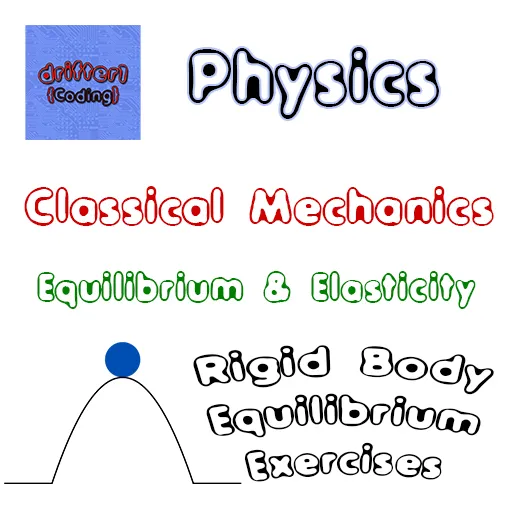[Custom Thumbnail that contains Image1]

Introduction

Hey it's a me again @drifter1! Today we continue with Physics and more specifically the branch of "Classical Mechanics". More specifically, in this penultimate article of Equilibrium and Elasticity we will cover Rigid Body Equilibrium Exercises. So, without further ado, let's dive straight into it!

Center of Gravity Calculation

Two-body system

Let's suppose that a sphere of radius r1 = 0.1 m and mass m1 = 1.00 Kg is connected to another sphere of radius r2 = 0.3 m and mass m2 = 2.00 Kg by a rigid rod of negligible mass (weightless) and length of 0.5m.

Where is the center of gravity of each sphere and the two-body system located at?

The mass of the spheres is uniformly distributed.

Solution

Because the mass of each sphere is uniformly distributed across the whole body, the center of mass is located at the geometrical center of the sphere. Because the mass is uniformly distributed the center of gravity is also at the same location as the center of mass.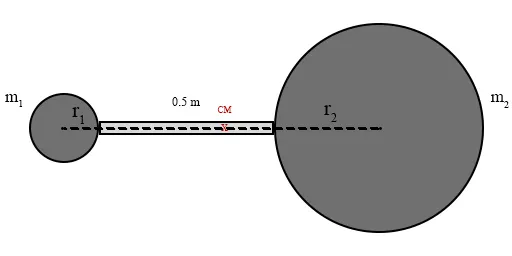[Custom Figure]

Assuming that the mass of each sphere is concentrated at the center of mass/gravity, the center of mass/gravity between the two spheres will be somewhere along the line that connects those two points. Because the second sphere has a larger mass (double the mass), we expect the CoG to be closer to the second sphere.

Let's use the x axis along the dashed line as our coordinate system. Let's also define the distance of sphere 1 to the center of mass as R1, and in the same way, the distance of sphere 2 to the CoM as R2. Thus, the center of mass is at the point where: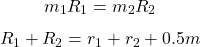Solving the system and defining R = r1 + r2 + 0.5m we get: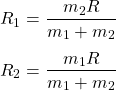Let's calculate the distances:So, the center of gravity is 0.3m left from the center of sphere 2 and 0.6m right from the center of sphere 1. Or we could also say that its exactly at the outer edge of sphere 2.

Three-body system

Let's suppose the same system as before, but with one change: The rod has now a uniform mass of m3 = 1 Kg.

Where is the center of gravity now?

Solution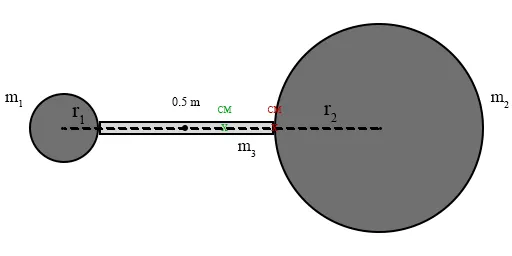[Custom Figure]

The center of mass/gravity of the rod is at its center and so 0.25m away from each sphere's edge. Instead of calculating the CoG for all three bodies together, we can use the previously calculated CoG and simply "attach" the new mass to it. By that I mean that the new CoG will be somewhere between the added mass and the "original" CoG.

The center of gravity is at the point where: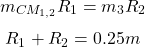Solving the system we get that:Therefore, the center of gravity of the three-body system is 0.0625m to the right of the center of the rod, and 0.1875m to the left of previous center of mass (the edge of the second sphere).

Equilibrium Problems

Torque Balancing (simplified version of Example 12.3 of Reference 3)

Three masses are attached to a meter stick with negligible mass and balancing on top of a fulcrum, as shown in the Figure below. The masses m1 = 50 g and m2 = 100 g are to the left of the fulcrum. Find the mass m3 that balances the system when it is attached at the right end of the stick, and the normal reaction force at the fulcrum when the system is balanced.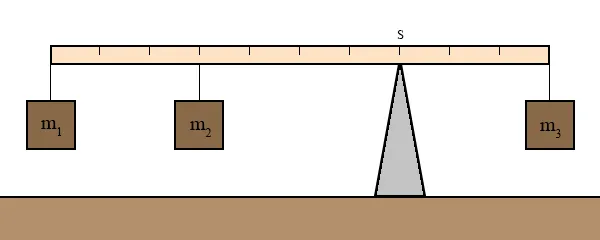[Custom Figure based on Figure 12.9 of Reference 3]

Solution

We can identify that a total of four forces are acting upon the meter stick: The weight of each of the masses and the normal reaction force FS at the support point S. All the forces are acting on the verital or y-axis, which is the direction of gravity. The pivot point of rotation is at point S, and so the axis of rotation is perpendicular to the x-axis and passing through that point.

Let's draw a free-body diagram for the meter stick:
[Custom Figure based on Figure 12.10 of Reference 3]

Let's first calculate the distance of each force from point S.Considering that counterclockwise rotation is causing positive torque, we now write each of the four forces in respect to the chosen pivot S.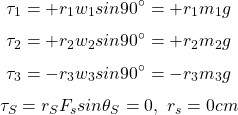Now that we defined all the forces and torques that act upon the meter stick, we can write the first and second equilibrium conditions and then solve the system.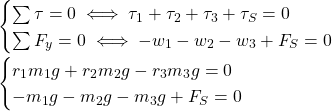We end up with a system of two equations with unknown values m3 and FS. Because the first equation doesn't contain FS we can direclty solve for m3 by cancelling the g, rearranging the terms and dividing by r3:So, the mass of the right-most object is m3 = 250g.

Solving the second equation for FS, we can now calculate the normal reaction force of the fulcrum.Thus, the reaction force is FS = 3.92 N.

Ladder Resting Against a Wall (based on Example 12.5 of Reference 3)

A uniform ladder of length L and weight w rests against a slippery vertical wall, as shown in the Figure that follows. The angle between the ladder and the rough floor is θ. Calculate the reaction forces from the floor and from the wall on the ladder, as well as the coefficient of static friction μS at the interface of the ladder with the floor that prevents the ladder from slipping.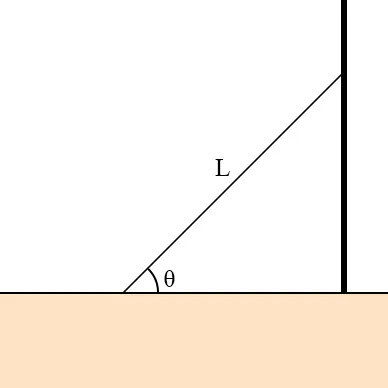[Custom Figure based on Figure 12.14 of Reference 3]

Solution

Four forces act upon the ladder:

1. The normal reaction force N from the floor to the ladder in the upward vertical direction
2. The static friction force f = μS N directed horizontally along the floor and towards the wall (preventing the ladder from slipping)
3. The weight w of the ladder, attached at its CoG thats in the middle of its ends
4. The normal reaction force F from the wall to the ladder in the horizontal direction away from the wall
The wall is slippery and so there is no friction between the wall and ladder.

Let's draw a free-body diagram for the ladder: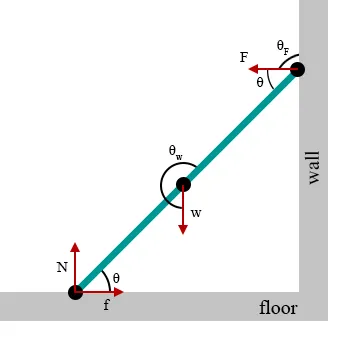[Custom Figure based on Figure 12.15 of Reference 3]

From the free-body diagram, the net forces in the x and y directions are:Choosing the contact point with the floor as the pivot point of rotation, the forces N and f have zero torque, and thus the net torque is: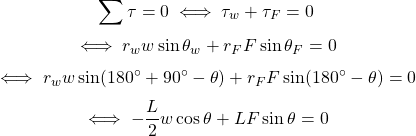This final equation can now be solved for F giving us: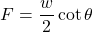From F = f and N = w, the coefficient of static friction is: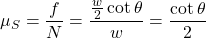The net force on the ladder at the contact point with the floor is equal to the vector sum of N and f, which are perpendicular to each other and thus their magnitude is: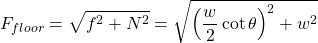and its direction is: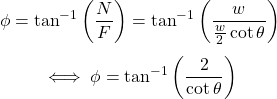We conclude that the reaction forces F from the wall, and Ffloor from the floor, as well as the coefficient μS, are only related to the weight of the ladder w and the angle θ. Its also quite interesting to node that the results are independent of the length of the ladder L as its cancelled in the second equilibrium condition. The ladder will slip when the net torque becomes negative, which happens when the coefficient of static friction is not great enouigh to prevent the ladder from slipping.

RESOURCES:

Images

1.Final words | Next up

And this is actually it for today's post!

Next time we will get into Elasticity and Plasticity Exercises...

See ya!Keep on drifting!
H2
H3
H4
3 columns
2 columns
1 column
1 Comment